Register for Maths, Science, English, Reasoning Olympiad Exams Register here | Book Free Demo for Live Olympiad Classes here | Check Olympiad Exam Dates here | Buy Practice Papers for IMO, IOM, HEO, IOEL etc here | Login here to participate in all India free mock test on every Saturday

# Ratio and Proportion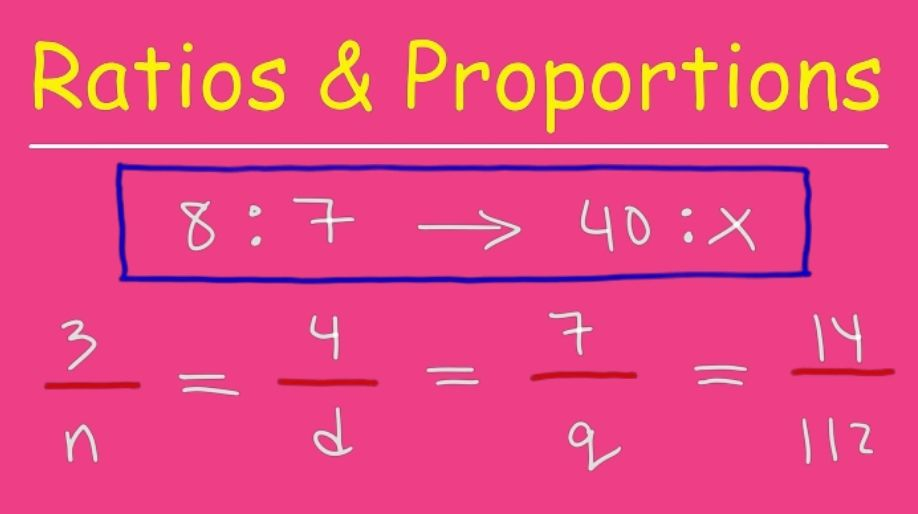What is ratio and proportion? We all come across various kinds of comparisons in each and every field. We do the comparison by how many times or by how much? Here we will learn more about the above questions.

In mathematics, we define a ratio as a relationship between two numbers indicating how many times the first number contains the second number.

In simple words we can say that a ratio is a comparison between two numbers which tells us that first number is how many times the second number.

For example the cost of an apple is Rs 30 whereas the cost of an orange is Rs 15 .Hence the cost of an apple is twice the cost of an orange. Hence the ratio is two and it is represented as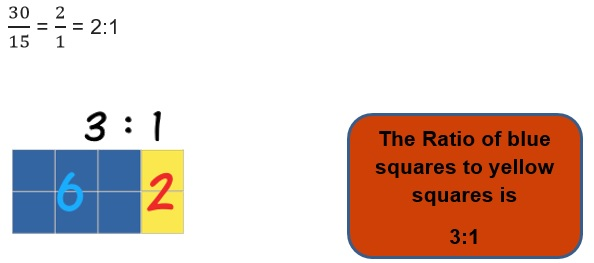Here an important is to be remembered that only those quantities can be compared which has the same unit or they are in the same unit.

For example the price of a pencil and pen can be compared but the price of the pencil cannot be compared to the weight of the pen.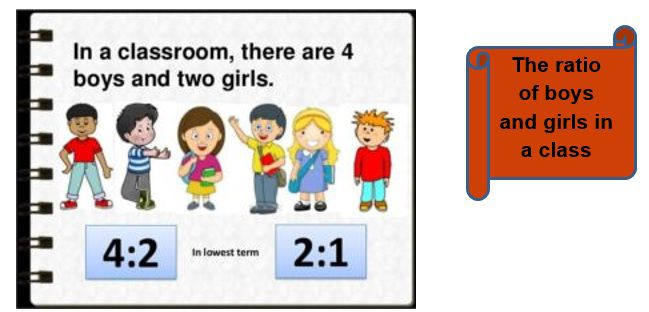Example 1: The length of a park is 120m the breadth is 45m. Find the ratio of length to breadth and also find the ratio of length to the area.

Solution: The ratio of length to breadth will be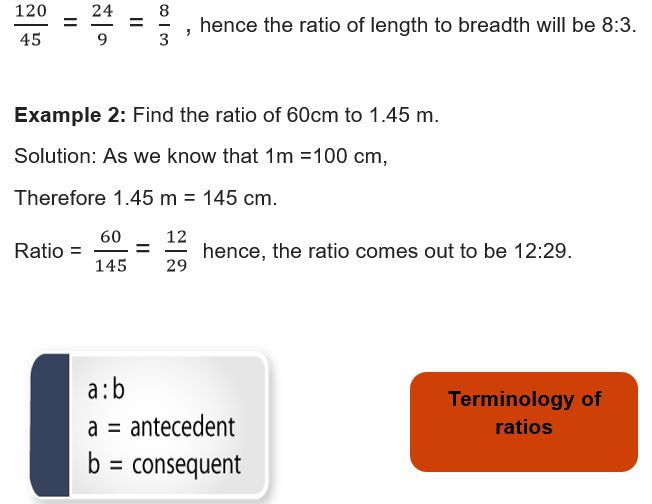Proportion

A proportion is a name which we give to any statement that two ratios are equal. It can be written in two ways, two equal fractions, or, using a colon, a: b = c: d or a: b::c:d.

• It is obvious that if the two ratios are not equal then they are definitely not in proportion.
• In any proportion the first and fourth term are called as extreme terms and the second and third as middle terms.

Example 3: Are the terms 20, 30, 40, 60 in proportion?

Solution: The ratio of first two terms is 20:30 = 2:3.

The ratio of the next two terms is 40:30 = 4:6= 2:3.

Since both the ratios are equal and hence the terms are in proportion.

Unitary Method

The method in which we first find out the value of one unit and then the value of required number of units is called as Unitary method.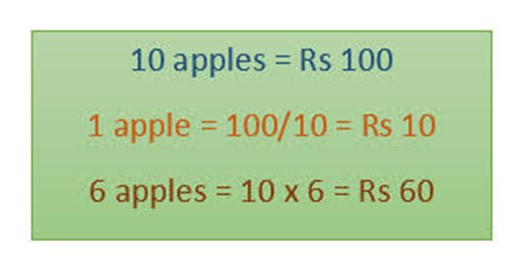Example 4: If the cost of 6 notebooks is Rs 72. Then what will be the cost of 4 notebooks?

Solution: Cost of 6 notebooks = Rs 72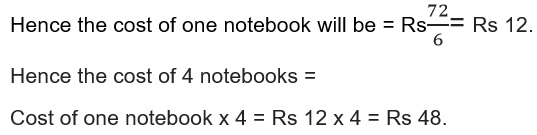Practice Questions

Q1) Ramesh made 63 runs in 7 overs and Raju made 72 runs in 8 overs. Who made more run per over?

Q2) The cost of 4kg wheat is Rs 48. Find the cost of 10 kg of wheat.

Q3) State True or False

1. a) Rs 40: Rs 200 = 80kg : 400kg
2. b) 23 litre : 69 litre = 12g:36g

Q4) Determine the proportion

1. 30, 40, 90, 360.
2. 1,2,4,8
3. 4,6,8,12

Q5) The cost of 5 dozen bananas is Rs 60.Find how much dozens of bananas one will get for Rs 40.

Recap

• For comparison by ratio, the two quantities must be in the same unit. If they are not, they should be expressed in the same unit before the ratio is taken.

• The order in which quantities are taken to express their ratio is important.

• Two ratios are equivalent, if the fractions corresponding to them are equivalent. Thus, 3 : 2 is equivalent to 6 : 4 or 12:8.

• The method in which we first find the value of one unit and then the value of the required number of units is known as the unitary method.

• In ratio and proportion the order is very important because as the order changes the ratio also changes.

## Quiz for Ratio and Proportion

 Q.1 Unit of ratio a) m b) m/s² c) kg d) none of the above
 Q.2 What is the most important character that signifies that the number is a ratio? a) . b) : c) @ d) *
 Q.3 The value of {-8/13 × 26/-3} is _____ a) -8/13 b) 7/26 c) -4/13 d) 16/3
 Q.4 To find the ratio of two numbers it is necessary that: a) The numbers should have unequal units b) The numbers should be equal c) The numbers have the same unit d) None of the above
 Q.5 The product of two numbers is -20/9. If one of the numbers is 4, find the other. a) –5/9 b) 3/11 c) 12/39 d) –9/11
 Q.6 Fill in the blank: - 19:25___25:19. a) :: b) ₌ c) ≠ d) none of the above
 Q.7 Express 19kg and ₹38 in a ratio. a) 19:38 b) 1:02 c) 38:19 d) Cannot be expressed as a ratio.
 Q.8 Express 8m and 6m in a ratio. a) 4:03 b) 8:06 c) 6:08 d) 3:04
 Q.9 Find the ratio of 8 months to 3 years. a) 3:8 b) 8:3 c) 9:2 d) 2:9
 Q.10 A man earns 200 as his daily earnings. He spends 20/- on his food, he gives 15/- to his wife, he puts 60/- in his savings, and the rest he spends on daily expenditure. Calculate the ratio of money spent on food. a) 1:10 b) 3:40 c) 3:10 d) 21:40